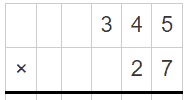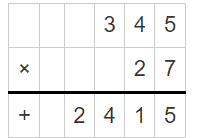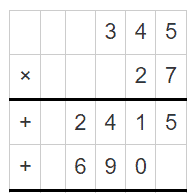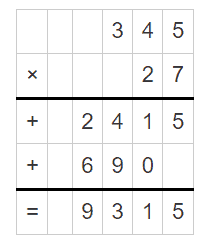# Long Multiplication Worksheets

• ###### Long Multiplication #15

Long multiplication is a method used to multiply two or more numbers with multiple digits. It involves multiplying the digits of one number by each digit of the other number and then summing up the results. Long multiplication is typically taught in elementary or middle school mathematics curricula.

These worksheets consist of a series of multiplication problems, each involving two or more numbers with multiple digits. The problems are arranged in a structured format, with one number placed above the other, similar to how the multiplication is done manually. Students are expected to multiply each digit of the bottom number by the digits of the top number, starting from the rightmost digit, and then add up the partial products to obtain the final product. They are also set in a matrix which provides an easy way for students to determine the proper place value columns.

These worksheets can vary in complexity, starting from simpler problems with two-digit numbers and progressing to more challenging ones with larger numbers. They also include additional features, such as carrying over digits during multiplication or incorporating word problems to apply long multiplication in real-life scenarios.

By practicing with long multiplication worksheets, students develop their skills in multiplication, reinforce their understanding of place value, improve their ability to perform calculations accurately, and enhance their overall numerical fluency. These worksheets serve as valuable tools for educators, parents, and students to facilitate learning and mastery of long multiplication concepts.

### How Do You Do Long Multiplication?

Long multiplication is a method used to multiply two or more numbers with multiple digits. Here’s a step-by-step guide on how to perform long multiplication:

Let’s take an example: 345 × 27

Step 1: Write the numbers one above the other, lining up the digits based on their place values:Step 2: Multiply the digits in the ones place (rightmost column) of the bottom number (7) with each digit of the top number (345) and write the partial products below the line, shifted to the right:Step 3: Now, multiply the digit in the tens place (2) of the bottom number with each digit of the top number. Write the partial products shifted one place to the left compared to the previous step:Step 4: Add up the partial products:Step 5: The final result is 9315, which is the product of 345 and 27.

It’s important to remember to align the digits correctly and keep track of place values while performing long multiplication. Carrying over digits from one place value to the next may be required if the partial product exceeds 9.